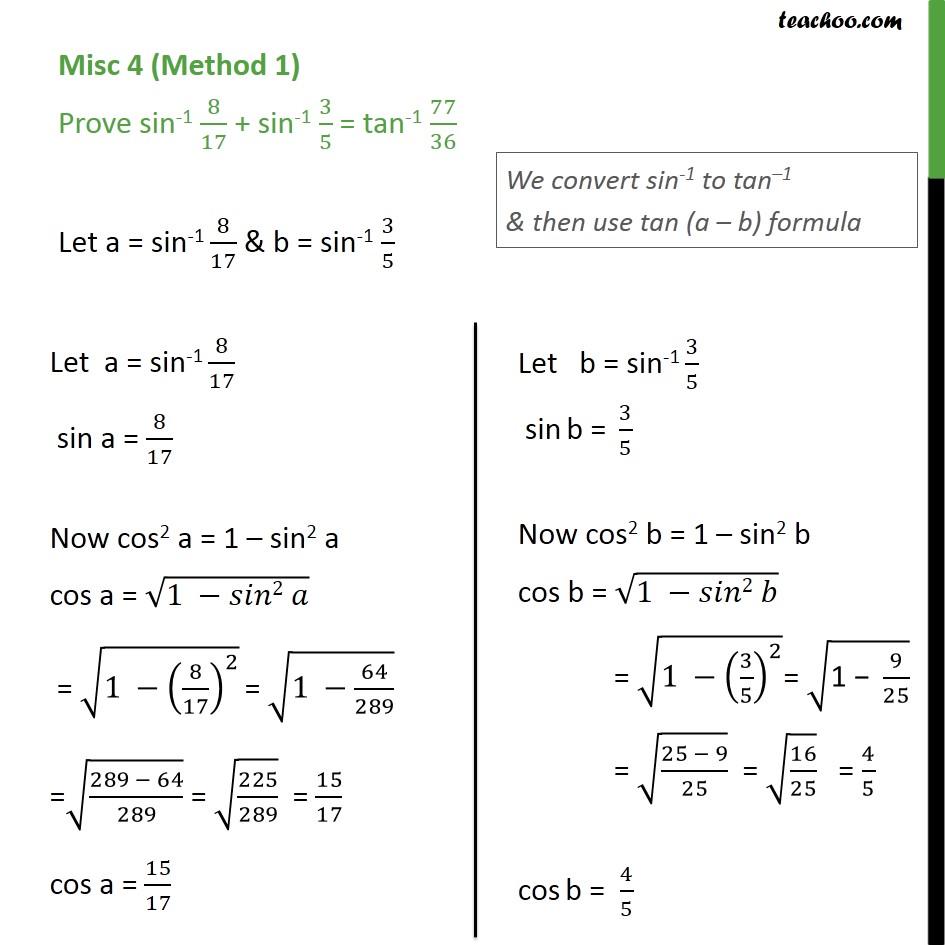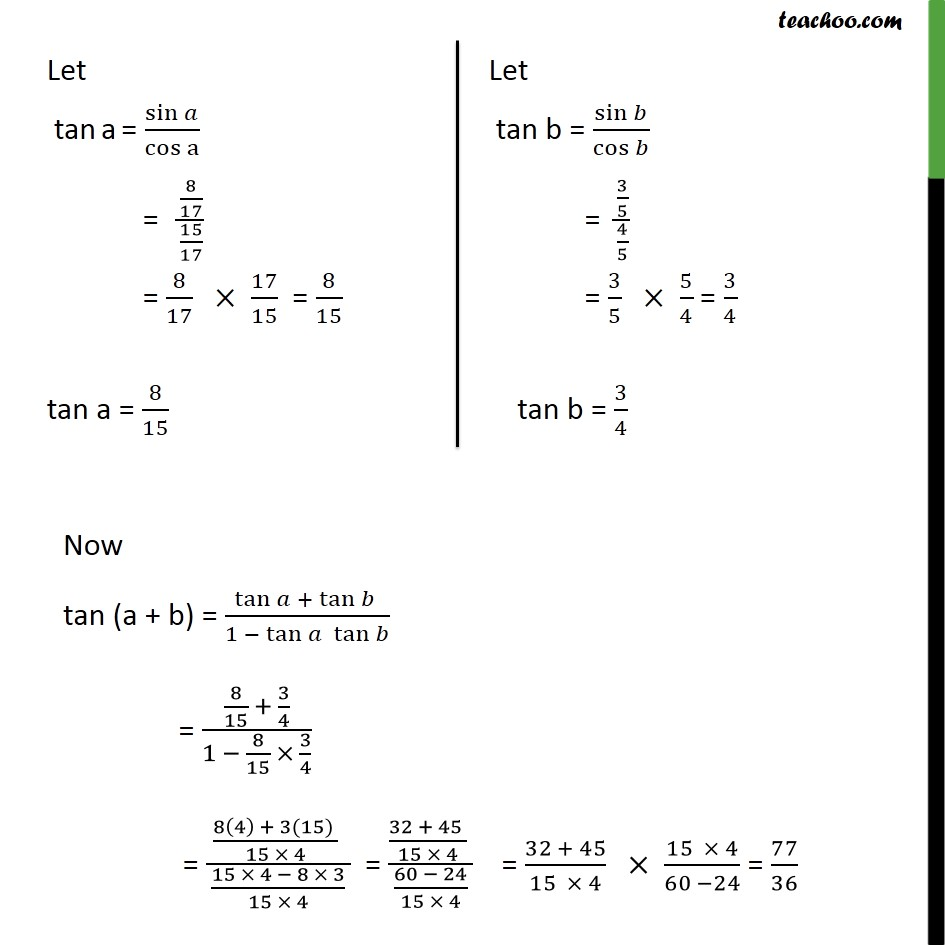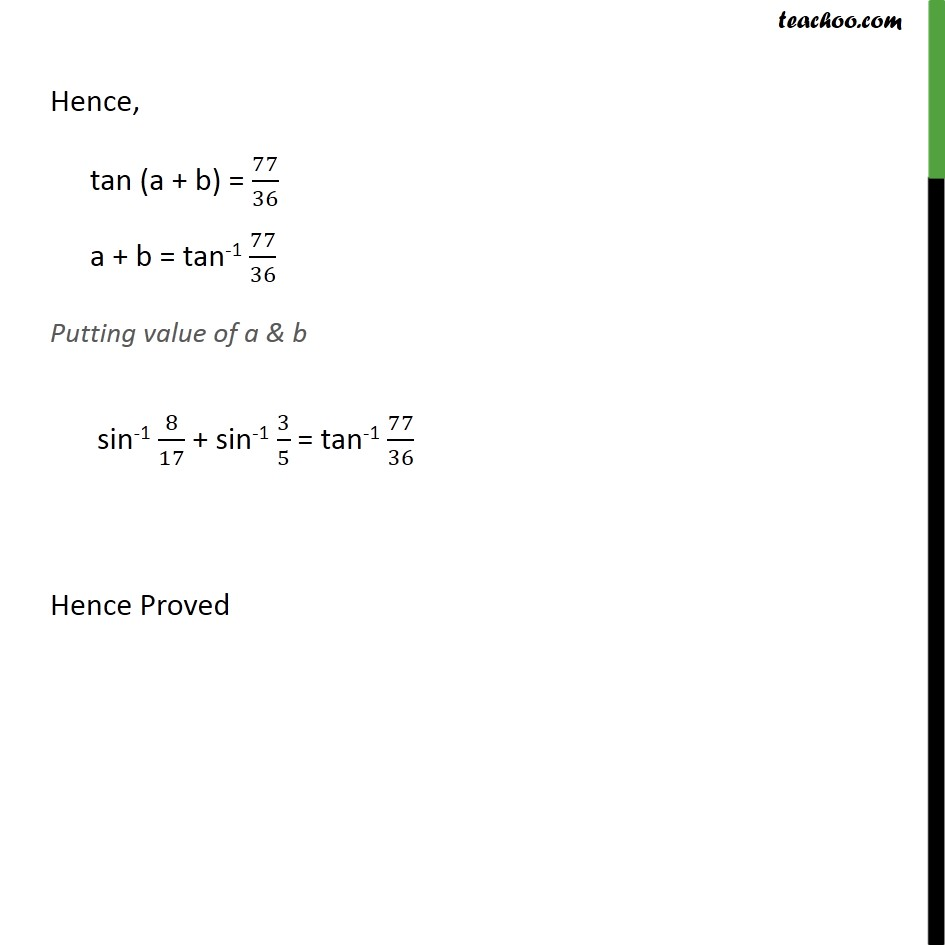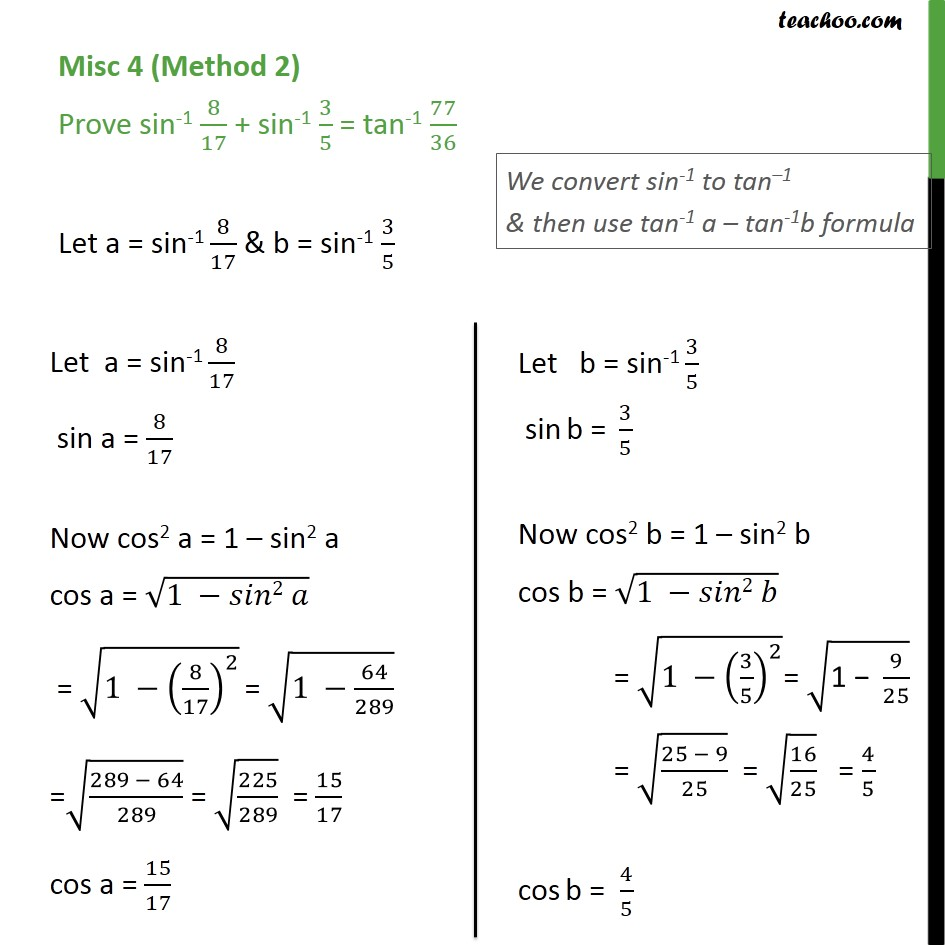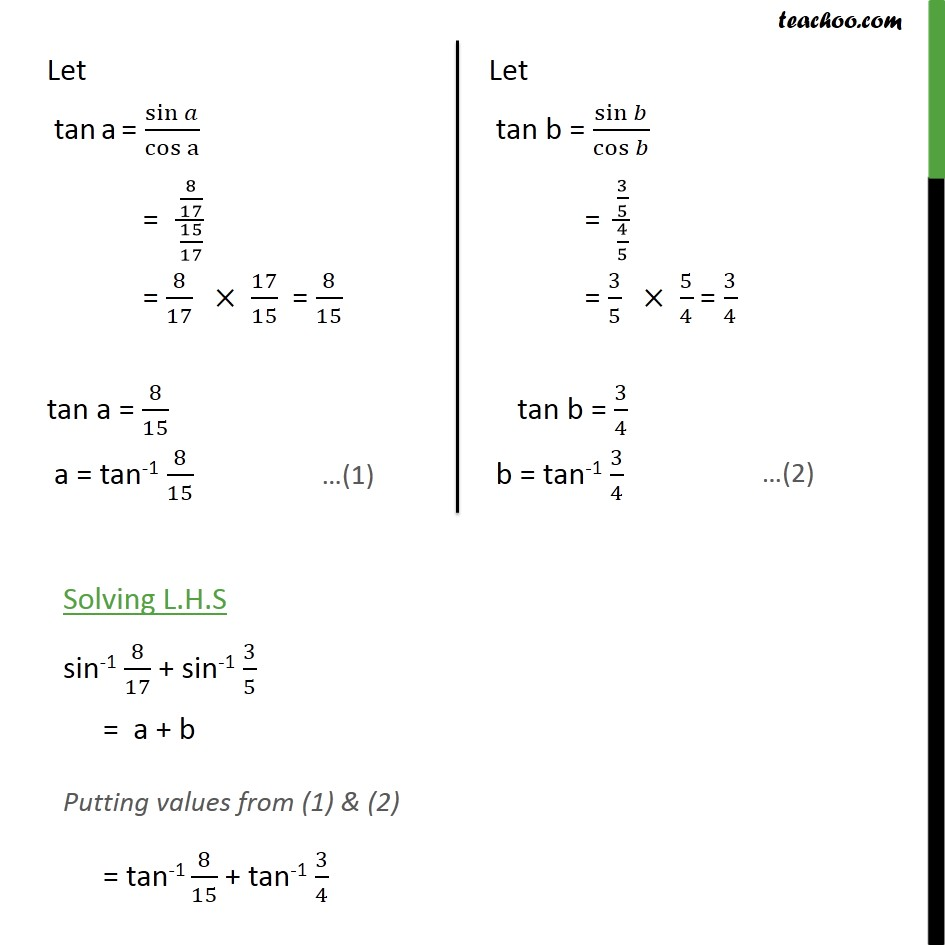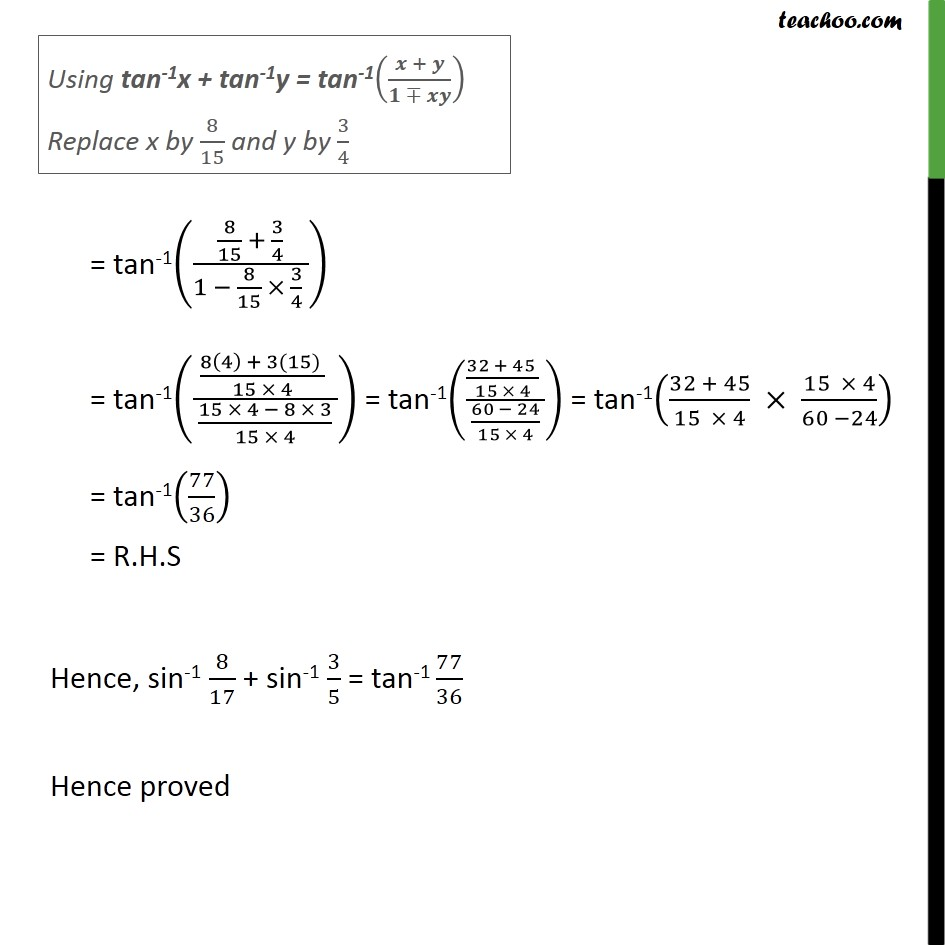1. Chapter 2 Class 12 Inverse Trigonometric Functions
2. Serial order wise
3. Miscellaneous

Transcript

Misc 4 (Method 1) Prove sin-1 8/17 + sin-1 3/5 = tan-1 77/36 Let a = sin-1 8/17 & b = sin-1 3/5 Now tan (a + b) = tan + tan /(1 tan tan ) = (8/15 + 3/4)/(1 8/15 3/4) = ((8(4) + 3(15) )/(15 4) )/( (15 4 8 3)/(15 4) ) = ((32 + 45 )/(15 4) )/( (60 24)/(15 4) ) = (32 + 45)/(15 4) (15 4)/(60 24) = 77/36 Hence, tan (a + b) = 77/36 a + b = tan-1 77/36 Putting value of a & b sin-1 8/17 + sin-1 3/5 = tan-1 77/36 Hence Proved Misc 4 (Method 2) Prove sin-1 8/17 + sin-1 3/5 = tan-1 77/36 Let a = sin-1 8/17 & b = sin-1 3/5 Solving L.H.S sin-1 8/17 + sin-1 3/5 = a + b Putting values from (1) & (2) = tan-1 8/15 + tan-1 3/4 = tan-1((8/15 + 3/4)/(1 8/15 3/4 )) = tan-1(((8(4) + 3(15) )/(15 4) )/( (15 4 8 3)/(15 4) )) = tan-1(((32 + 45 )/(15 4) )/( (60 24)/(15 4) )) = tan-1((32 + 45)/(15 4) (15 4)/(60 24)) = tan-1(77/36) = R.H.S Hence, sin-1 8/17 + sin-1 3/5 = tan-1 77/36 Hence proved

Miscellaneous